• #include <stdio.h> typedef struct ss{ char a;//偏移量为0，大小为1,0能被1整除，=1 short b;//偏移量为1，大小为2,1不能被2整除，=1+1+2=4 int c;//偏移量为4，大小为4,4能被4整除，=4+4=8 ...
#include <stdio.h>

typedef struct ss{
char a;//偏移量为0，大小为1,0能被1整除，=1
short b;//偏移量为1，大小为2,1不能被2整除，=1+1+2=4
int c;//偏移量为4，大小为4,4能被4整除，=4+4=8
long d;//偏移量为8，大小为4,8能被4整除，=8+4=12
} ss_t;
struct ss s1[]={
{0x1,0x2,0x3,0x4},
{0x5,0x6,0x7,0x8},
{0xa,0xb,0xc,0xd},
};
int s1_len= sizeof(s1)/sizeof(s1);
struct ss s2[]={
{0x1,0x2,0x3,0x4},
{0x5,0x6,0x7,0x8},
{0xa,0xb,0xc,0xd},
{},
};
int s2_len= sizeof(s2)/sizeof(s2);
struct ss s3[]={
{},
{0x1,0x2,0x3,0x4},
{0x5,0x6,0x7,0x8},
{0xa,0xb,0xc,0xd},
};
int s3_len= sizeof(s3)/sizeof(s3);
int main()
{
/* 我的第一个 C 程序 */
printf("sizeof(s1)=%d, szieof(s1)=%d, s1_len=%d \n", sizeof(s1), sizeof(s1), s1_len);
printf("sizeof(s2)=%d, szieof(s2)=%d, s2_len=%d \n", sizeof(s2), sizeof(s2), s2_len);
printf("sizeof(s3)=%d, szieof(s3)=%d, s3_len=%d \n", sizeof(s3), sizeof(s3), s3_len);
return 0;
}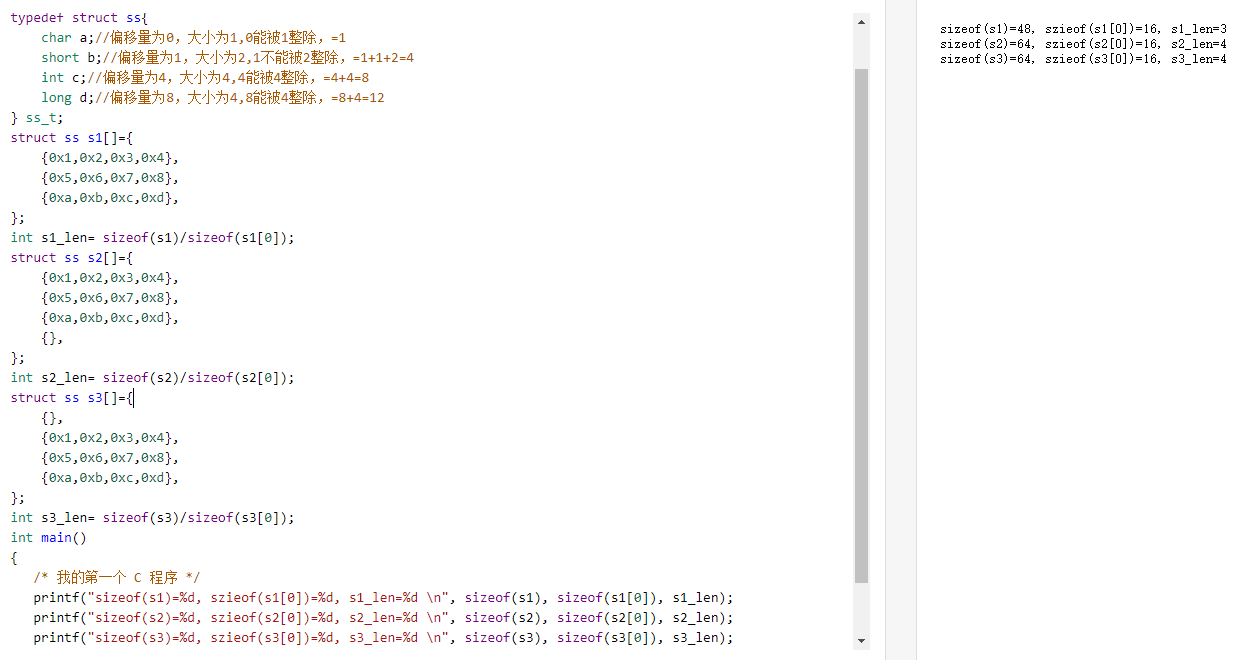展开全文• 先看一下：C/C++变量做数组长度 用MFC做抓猴子的小游戏，用同样的方法在头文件中定义结构体数组报错。 protected: // create from serialization only CCatchMonkeyView(); DECLARE_DYNCREATE(CCatchMonkeyView) ...
先看一下C/C++定义可变数组的方法：[C/C++变量做数组长度]
(https://blog.csdn.net/lyq_12/article/details/81773601) 用MFC做抓猴子的小游戏，用同样的方法在头文件中定义结构体数组报错。
protected: // create from serialization only
CCatchMonkeyView();
DECLARE_DYNCREATE(CCatchMonkeyView)

// Attributes
public:
CCatchMonkeyDoc* GetDocument();
int sum;	//猴子总数
int remain;	//剩余猴子数
int height;
int width;

struct MONKEY{
float x;
float y;
bool isCatched;
};

MONKEY* monkey;
monkey = new MONKEY[sum];	//通过指针动态申请空间

报错信息
error C2327: 'CCatchMonkeyView::sum' : member from enclosing class is not a type name, static, or enumerator
error C2258: illegal pure syntax, must be '= 0'

问题出在不能在头文件中给数组分配空间，申请空间的操作应该在构造函数初始化时候完成。
正确姿势 在头文件中声明，构造函数中分配空间。
public:
CCatchMonkeyDoc* GetDocument();
int sum;	//猴子总数
int remain;	//剩余猴子数
int height;
int width;

struct MONKEY{
float x;
float y;
bool isCatched;
};

MONKEY* monkey;



CCatchMonkeyView::CCatchMonkeyView()
{
// TODO: add construction code here
width=height=0;
sum=10;

monkey = new MONKEY[sum];	//通过指针动态申请空间

remain=0;
for(int i=0;i<sum;i++){
monkey[i].x=rand()%800+1;
monkey[i].y=rand()%450+1;
monkey[i].isCatched=false;
}
srand((unsigned)time(NULL));
}

展开全文c++ c语言 mfc
• 结构体数组 结构体数组格式：声明变量类型 数组名称[数组长度] //定义一个结构体数组名为a,它里面包含有5个元素，每一个元素都是一个结构体变量 Wor a={}; ...
结构体数组
结构体数组的定义：
结构体数组格式：声明变量类型 数组名称[数组长度]
//定义一个结构体数组名为a,它里面包含有5个元素，每一个元素都是一个结构体变量
Wor a={};

#include<stdio.h>
typedef struct worker{
char id;
char name;
char sex;
int pay;
}WOR;
void main(){
WOR a=
{
{"0001","陈文杰","男",1200},
{"0001","张三丰","女",500},
{"0001","张四丰","女",1200}
}
}

对比记忆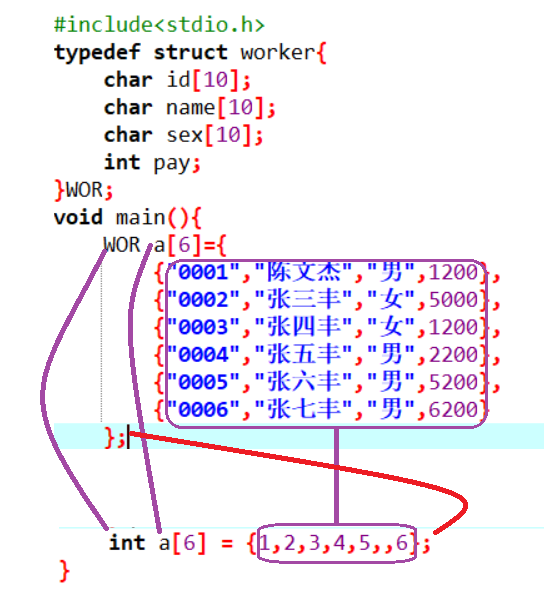结构体数组的输出
#include<stdio.h>
typedef struct worker{
char id;
char name;
char sex;
int pay;
}WOR;
void main(){
WOR a={
{"0001","陈文杰","男",1200},
{"0002","张三丰","女",5000},
{"0003","张四丰","女",1200},
{"0004","张五丰","男",2200},
{"0005","张六丰","男",5200},
{"0006","张七丰","男",6200}
};
int i;
for(i=0;i<6;i++){
printf("%s,%s,%s,%d\n",a[i].id,a[i].name,a[i].sex,a[i].pay);
}
}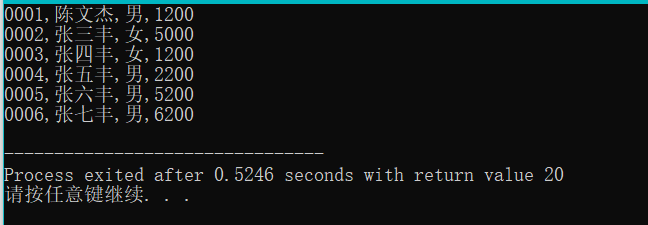结构体对象中的a[i]就代表一个对象，它已经不再是一个值了 所以我们应该用a[ii].成员名
改进以上程序
#include<stdio.h>
typedef struct worker{
char id;
char name;
char sex;
int pay;
}WOR;
void output(WOR a[],int n){//次函数是用来输出长度为n的工人数组的信息
int i;
printf("工号\t姓名\t性别\t年龄\n");
for(i=0;i<6;i++){
printf("%s\t%s\t%s\t%d\n",a[i].id,a[i].name,a[i].sex,a[i].pay);
}
}
void main(){
WOR a={
{"0001","陈文杰","男",1200},
{"0002","张三丰","女",5000},
{"0003","张四丰","女",1200},
{"0004","张五丰","男",2200},
{"0005","张六丰","男",5200},
{"0006","张七丰","男",6200}
};
output(a,6);
}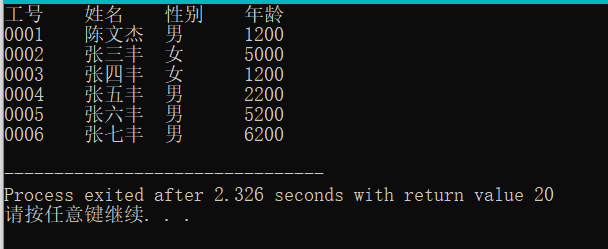结构体的应用
案例一、
1、编写一个函数，它的目的是为了输出所有性别为男的的职工信息 算法分析： 1)这个函数它不用返回值因为该函数是为了完成输出 2）该函数要处理很多职工，就需要一个数组，数组的类型为职工类型 注意：在C语言当中字符串不能直接比较是否相同，要通过strcmp这个函数来比较其两个字符串是否相同
#include<stdio.h>
#include<string.h>
typedef struct worker{
char id;
char name;
char sex;
int pay;
}WOR;
void QueryWorker(WOR a[],int n){//次函数是用来输出长度为n的工人数组的信息
int i;
printf("全部员工：\n");
printf("工号\t姓名\t性别\t年龄\n");
for(i=0;i<6;i++){
printf("%s\t%s\t%s\t%d\n",a[i].id,a[i].name,a[i].sex,a[i].pay);
}
printf("所有男员工：\n");
printf("工号\t姓名\t性别\t年龄\n");
for(i=0;i<6;i++){
if(strcmp(a[i].sex,"男")==0){
printf("%s\t%s\t%s\t%d\n",a[i].id,a[i].name,a[i].sex,a[i].pay);
}
}
printf("所有女员工：\n");
printf("工号\t姓名\t性别\t年龄\n");
for(i=0;i<6;i++){
if(strcmp(a[i].sex,"女")==0){
printf("%s\t%s\t%s\t%d\n",a[i].id,a[i].name,a[i].sex,a[i].pay);
}
}
}
void main(){
WOR a={
{"0001","陈文杰","男",1200},
{"0002","张三丰","女",5000},
{"0003","张四丰","女",1200},
{"0004","张五丰","男",2200},
{"0005","张六丰","男",5200},
{"0006","张七丰","男",6200}
};
QueryWorker(a,6);
}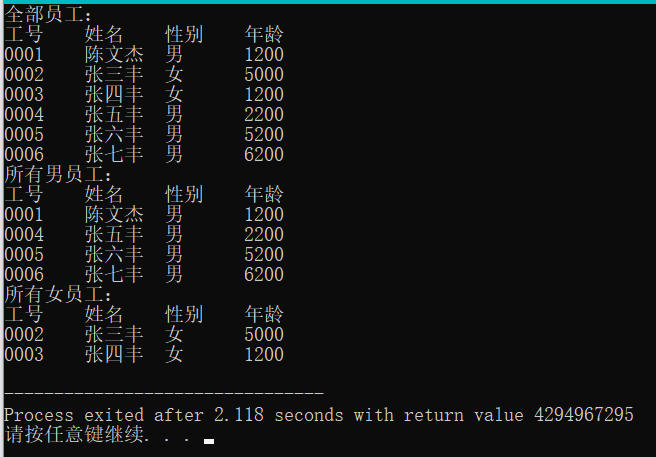2、能够输出指定性别的职工
#include<stdio.h>
typedef struct worker{
char id;
char name;
char sex;
int pay;
}WOR;
void QueryBySex(WOR a[],int n,char b[]){//次函数是用来输出长度为n的工人数组的信息
int i;

printf("工号\t姓名\t性别\t年龄\n");
for(i=0;i<6;i++){
if(strcmp(a[i].sex,b)==0){
printf("%s\t%s\t%s\t%d\n",a[i].id,a[i].name,a[i].sex,a[i].pay);
}
}

}
void main(){
WOR a={
{"0001","陈文杰","男",1200},
{"0002","张三丰","女",5000},
{"0003","张四丰","女",1200},
{"0004","张五丰","男",2200},
{"0005","张六丰","男",5200},
{"0006","张七丰","男",6200}
};
char b;
printf("请输入您要查询的性别 :\n");
scanf("%s",b);
QueryBySex(a,6,b);
}

案例二、
让用户输入一个工号，然后在一个结构体数组当中去查找有没有这个工人，如果有则输出这个工人的信息，如果没有则找不到此人
#include<stdio.h>
typedef struct worker{
char id;
char name;
char sex;
int pay;
}WOR;

void FindById(WOR a[],int n,char id[]){
//我们要访问数组里面每一个工人，如果发现了一个工人，就退出循环。如果找不到就输出找不到此人
int i;
for(i=0;i<n;i++){
if(strcmp(a[i].id,id)==0){//说明第i个工人就是我们要找的职工
break;//强制终止循环
}
}

//在退出循环之后，再来检查i与n的关系
if(i<n){//说明循环在中途就break,发现了职工
printf("%s\t%s\t%s\t%d\n",a[i].id,a[i].name,a[i].sex,a[i].pay);
}
else//找不到职工
{
printf("找不到此人");
}
}

void main(){
WOR a={
{"0001","陈文杰","男",1200},
{"0002","张三丰","女",5000},
{"0003","张四丰","女",1200},
{"0004","张五丰","男",2200},
{"0005","张六丰","男",5200},
{"0006","张七丰","男",6200}
};
char b;
printf("请输入您要查询的id :\n");
gets(b);
FindById(a,6,b);
}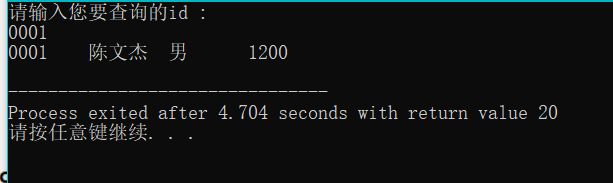案例三、
求出所有职工中最高工资那个职工的信息 注意：这里有一个知识点：成员比较整体赋值
#include<stdio.h>
#include<string.h>
typedef struct worker{
char id;
char name;
char sex;
int pay;
}WOR;
void max(WOR a[],int n){
WOR m=a;
int i;
for(i=0;i<n;i++){
if(m.pay<a[i].pay){//如果m的工资小于a[i]的工资，就把m赋值给a[i]
m=a[i];
}
}
printf("%s\t%s\t%s\t%d\n",m.id,m.name,m.sex,m.pay);
//假设第一个工人是最高工资的那个工人
}
void main(){
WOR a={
{"0001","陈文杰","男",1200},
{"0002","张三丰","女",7000},
{"0003","张四丰","女",1200},
{"0004","张五丰","男",2200},
{"0005","张六丰","男",5200},
{"0006","张七丰","男",6200}
};
max(a,6);
}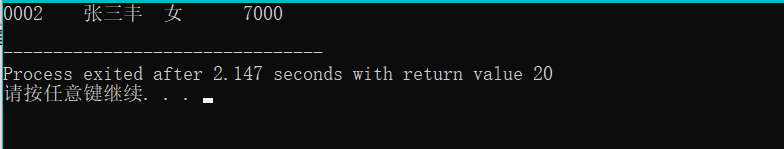上方程序改进 输出所有工资大于平均工资的职工 1、必须要先求出平均 2、在访问每一个职工，如果发现这个职工的工资要小于平均工资的话，就输出这个职工的信息。
#include<stdio.h>
#include<string.h>
typedef struct worker{
char id;
char name;
char sex;
int pay;
}WOR;
void QueryByAver(WOR a[],int n){
int i;
float aver;
int sum = 0;
//求出总工资
for(i=0;i<n;i++){
sum+=a[i].pay;
}
//求出平均工资aver
aver =(float)sum/n;
printf("平均工资为%f\n",aver);
printf("大于平均工资为：\n");
//访问每一个职工
for(i=0;i<n;i++){
if(a[i].pay>=aver){//如果发现职工的工资大于等于平局工资，就输出对应职工的信息
printf("%s\t%s\t%s\t%d\n",a[i].id,a[i].name,a[i].sex,a[i].pay);
}
}
}
void main(){
WOR a={
{"0001","陈文杰","男",3200},
{"0002","张三丰","女",7000},
{"0003","张四丰","女",1200},
{"0004","张五丰","男",2200},
{"0005","张六丰","男",5200},
{"0006","张七丰","男",6200}
};
QueryByAver(a,6);
}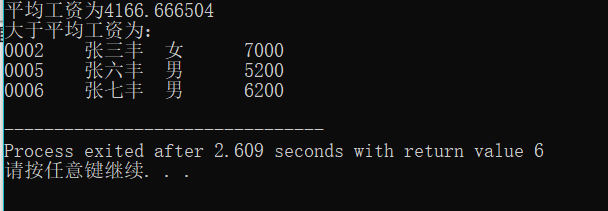算法变换
#include<stdio.h>
#include<string.h>
typedef struct stud{//定义了一个结构体
char Id;//学号
char Name;//姓名
int China;//语文成绩
int Math;//数学成绩
int English;//英语成绩
}STU;
//编写一个函数，它的目的是为了输出所有学生的总分与平均分
void fun(STU a[],int n){
int sum=0,i;
printf("学号\t姓名\t语文\t数学\t英语\t总分\t平局分\n");
//要访问里面的每一个学生
for(i=0;i<n;i++){
//对于一个学生a[i]而言我们要来输出它的信息
printf("%s\t%s\t%d\t%d\t%d\t%d\t%.1f\n",
a[i].Id,a[i].Name,a[i].China,a[i].Math,a[i].English,
a[i].China+a[i].Math+a[i].English,
(a[i].China+a[i].Math+a[i].English)/3.0);
sum = a[i].China+a[i].Math+a[i].English;
}
}
void main(){
STU a={
{"S1","张一军",127,150,100},
{"S2","张二军",75,85,89},
{"S3","张三军",67,58,86},
{"S4","张四军",120,101,87},
{"S5","张五军",136,100,82},
{"S6","张六军",141,52,83},
{"S7","张七军",125,85,81},
{"S8","张八军",136,65,79}
};
fun(a,8);
}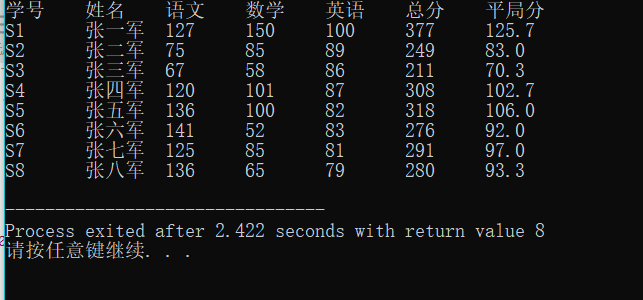案例变换： 注意字符串也不能够直接赋值，要通过一个函数来对字符串进行赋值 strcpy:通过这个函数才可以给字符串进行赋值
#include<stdio.h>
#include<string.h>
typedef struct stud{//定义了一个结构体
char Id;//学号
char Name;//姓名
int China;//语文成绩
int Math;//数学成绩
int English;//英语成绩
}STU;
//编写一个函数，求出每一个学生的优秀科目，目的不是输出分数，而是要输出对应科目的名称
void Perferct(STU a[],int n){
int sum=0,i;
char per;
int maxscore;//用来确保最优科目的分数
//要输出标题
printf("学号\t姓名\t语文\t数学\t英语\t最优秀科目\n");
//要访问里面的每一个学生，对于第i学生要确认它的最优科目名称（语文，数学，英语）
//科目名称是字符串，所以要先定义一个字符串来确保保存最优科目名称
for(i=0;i<n;i++){
maxscore=a[i].China;//认为第i个学生的语文是最优秀的
strcpy(per,"语文");
if(maxscore<a[i].Math){
maxscore = a[i].Math;
strcpy(per,"数学");
}
if(maxscore<a[i].English){
maxscore = a[i].Math;
strcpy(per,"英语");
}
printf("%s\t%s\t%d\t%d\t%d\t%s\n",
a[i].Id,a[i].Name,a[i].China,a[i].Math,a[i].English,per);
}
}

void main(){
STU a={
{"S1","张一军",127,150,100},
{"S2","张二军",75,85,89},
{"S3","张三军",67,58,86},
{"S4","张四军",120,101,87},
{"S5","张五军",136,100,82},
{"S6","张六军",141,52,83},
{"S7","张七军",125,85,81},
{"S8","张八军",136,65,79}
};
Perferct(a,8);
}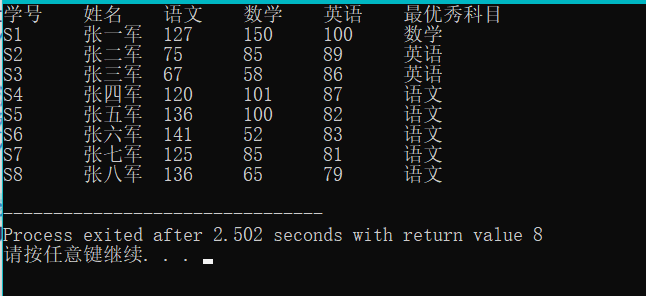展开全文c语言 程序设计 指针 编程语言
• 定义自定义类型的数组时，时常出现所占字长不一致的情形，需要用此办法解决
• C语言结构体数组+结构体类型指针+指向结构体数组的指针+typedef类型结构体数组两种结构体数组的定义形式用sizeof运算符求结构体数组所占字节数结构体类型指针指向结构体数组的指针typedef类型 结构体数组 如果要处理...
上一篇文章：C语言结构体类型定义+结构体变量的定义与使用及其初始化+结构体变量作为函数参数

C语言结构体数组+结构体类型指针+指向结构体数组的指针+typedef类型
结构体数组两种结构体数组的定义形式用sizeof运算符求结构体数组所占字节数结构体数组初始化结构体数组作为函数的参数查找符合条件的人数(一)

结构体类型指针 和 指向结构体数组的指针指向结构体变量的指针的定义利用结构体指针引用结构体变量成员指向结构体数组的指针查找符合条件的人数(二)
typedef类型学生成绩排序修改学生成绩下一篇文章

结构体数组
如果要处理多个类型相同的结构体数据，就需要定义结构体数组
两种结构体数组的定义形式
结构体数组定义的方法和结构体变量定义的方法一样，只不过加了一个数组的长度而已 第一种：和结构体类型同时定义，例如：
struct student
{
int sno;
char sname;
int sage;
char depmt;
float cscore;
}s1;//定义了一个结构体数组，数组名为s1，数组的长度为10

第二种：和结构体类型分开定义，如：
struct student
{
int sno;
char sname;
int sage;
char depmt;
float cscore;
};//不要忘记最后的分号
main()
{
struct student s1;//在主函数中定义结构体数组，数组名为s1，数组长度为10
...
}

用sizeof运算符求结构体数组所占字节数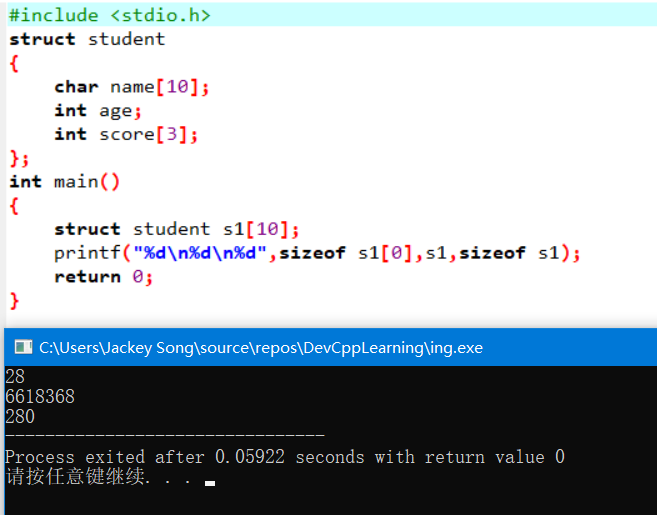char name 占用10个字节，int age 占用4个字节，int score占用3*4=12个字节，加起来总共是26个字节，可是上面输出的是28个字节，多出两个字节。这是因为在编译系统中，结构体类型存在字节边界的要求。而不同编译器字节边界的要求又是不一样的，上面这个案例我使用的是DevC++编译器，然而试验了一番之后，我并没能发现DevC++编译器字节边界要求的规律，反而把我给整懵圈了，如下：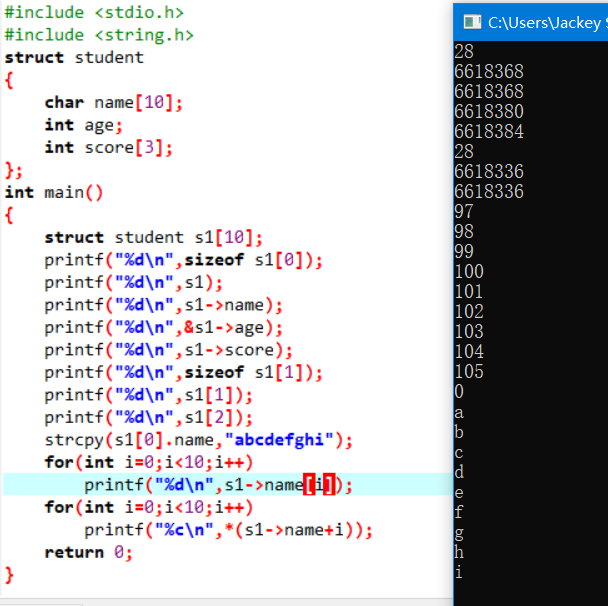虽然它的内存地址分配规律我没能搞懂，但是在使用的时候，依然可以正常用指针来操纵内存地址，进而操纵对应内存地址中的数据。 如下：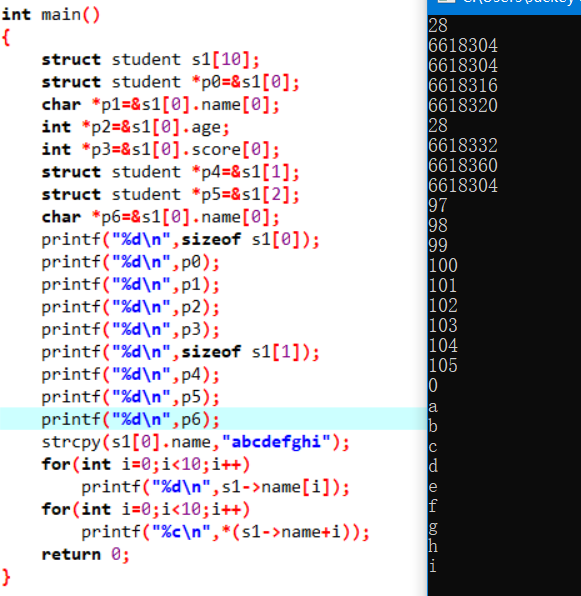结构体中的内存地址分配规律，相对于普通的数据类型的内存地址分配规律，要更加复杂一些，而且不同的编译器也不一样。 在VC编译器中，各成员变量在内存中的起始地址相对于结构体变量(或数组)的起始地址的偏移量，必须为该变量的类型所占字节数的倍数。如果某成员的偏移量不是该类型的整数倍，则VC编译系统会自动填充一定的字节数，以保证字节边界的要求。同时VC为了确保结构体的大小为结构体中所占内存空间最大的成员类型的字节数的倍数，在为最后一个成员变量申请空间后，还会根据需要自动填充空缺的字节。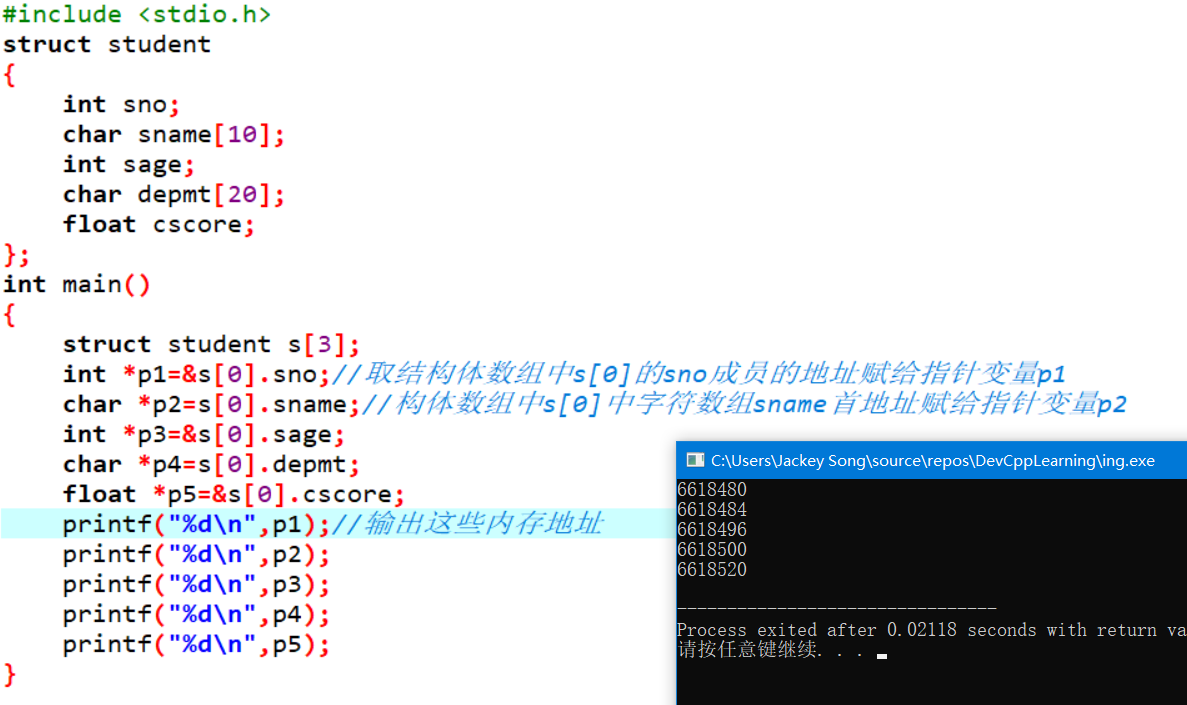内存地址相减得到各成员变量所占的字节数
注意： 访问结构体中的数据时，格式为结构体变量.结构体成员，这个时候使用的是点. 如果是一个指针变量p，p指向一个结构体类型，用指针来访问的话那么格式为指针变量->结构体成员，这个时候使用的是减号和大于号->，表示一个箭头，注意区分一下，使用的时候不要出错
结构体数组初始化
格式为：结构数组[n] = {{初值表1},{初值表2}, ... ,{初值表n}};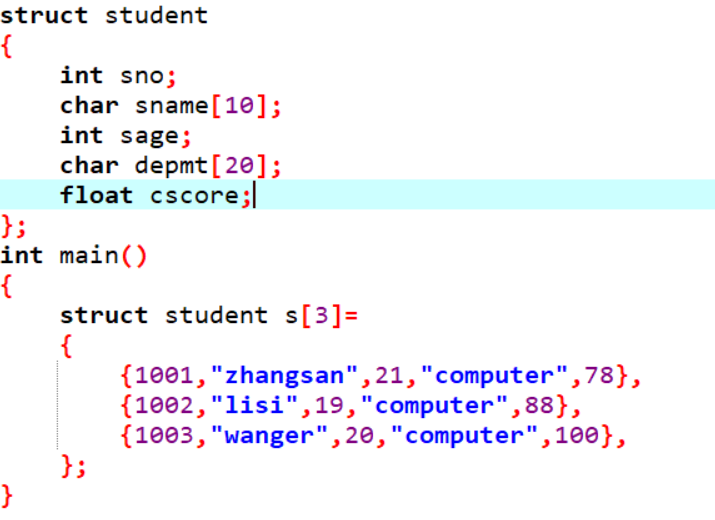用for循环输出结构体数组中的內容：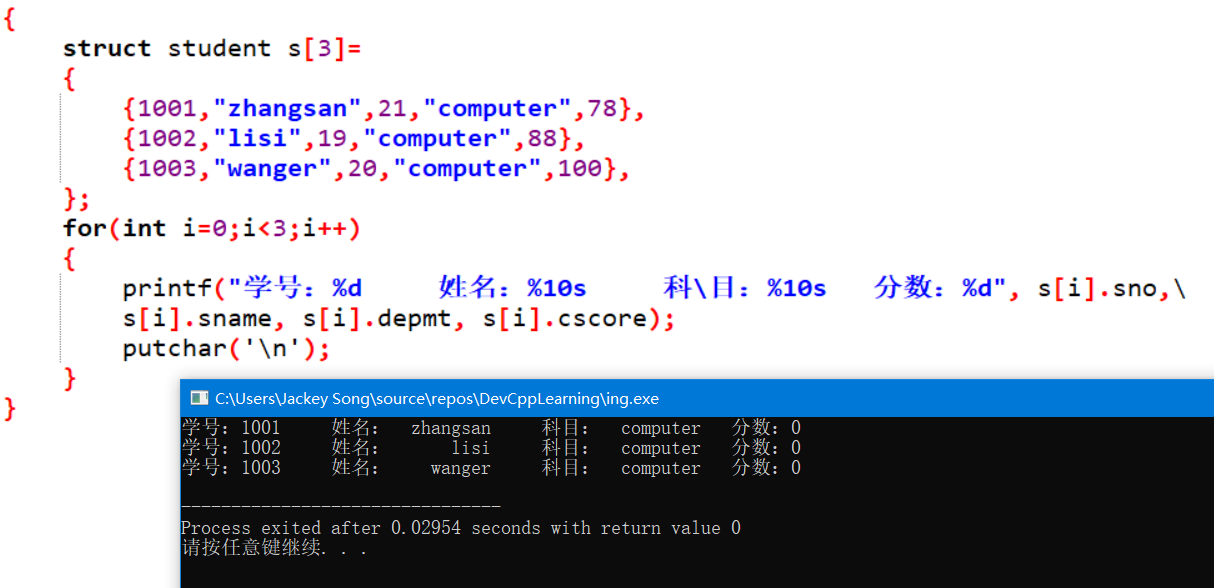当一个代码语句太长时，用反斜杠来进行代码换行 图中的代码有个字母打错了，导致最后的成绩输出全部是0，分数是float型的，而输出的时候却用了%d，应该用%f
结构体数组作为函数的参数
结构体数组作为函数的参数时，既可以作为形参，也可以作为实参，和普通数组一样。实参到形参的传递为地址传递。
查找符合条件的人数(一)
#include <stdio.h>
#define N 10
struct student
{
int no;
char name;
int score;
};
int fun(struct student s[],struct student b[],int m,int n);
void output(struct student x[],int n);
int main()
{
struct student s[N]=
{
{1001,"zhangsan",79},
{1002,"lisi",87},
{1003,"wanger",83},
{1004,"mazi",86},
{1005,"wangwu",89},
{1006,"wangliu",75},
{1007,"liwu",84},
{1008,"zhangwu",93},
{1009,"zhangqi",85},
{1010,"masan",97},
};
int m,n,k;
struct student a[N];
scanf("%d%d",&m,&n);
printf("The original data:\n");
output(s,N);
k=fun(s,a,m,n);
printf("The student that score in %d & %d is:\n",m,n);
output(a,k);
return 0;
}
int fun(struct student s[],struct student b[],int m,int n)
{
int i,k=0;
for(i=0;i<N;i++)
{
if(s[i].score>=m&&s[i].score<=n)
b[k++]=s[i];
}
return k;
}
void output(struct student x[],int n)
{
int i;
printf(" no          name       score\n");
for(i=0;i<n;i++)
printf("%d   %10s       %d\n",x[i].no,x[i].name,x[i].score);
putchar('\n');
}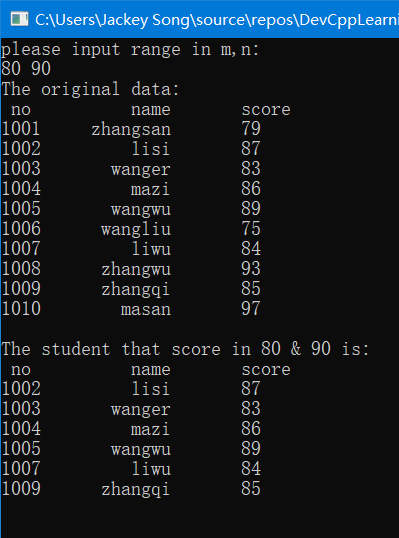结构体类型指针 和 指向结构体数组的指针
指向结构体变量的指针的定义
struct 结构体类型名 *指针名;
struct student stu;
struct student *ps=&stu;

利用结构体指针引用结构体变量成员
指针变量->结构体成员名; 取內容符*，*指针变量表示指针指向的变量， 也可以(*指针变量).成员名 所以有三种方法可以指向结构体成员变量： 结构体变量名 . 成员变量名 指向结构体变量的指针变量的名字 -> 成员变量名 (*指向结构体变量的指针变量的名字) . 成员变量名，括号不可省略
"."是分量运算符

指向结构体数组的指针
struct student s;
struct student *ps=s;//结构体变量名“s”为结构体数组的首地址

也可以写成：
struct student *ps=&s;

查找符合条件的人数(二)
结构体数组作为函数的实参时，可以用指针变量作为形参来接收结构体数组的内存地址，进而进行操作，把上面的代码改用指针来做，如下：(用指针做会更麻烦一些，但是为了加深对指针的印象和应用，我还是用指针做了一遍)
#include <stdio.h>
#define N 10
struct student
{
int no;
char name;
int score;
};
int fun(struct student *p,struct student *p2,int m,int n);//用指针来接收结构体数组的内存地址
void output(struct student *p,int n);//用指针来接收结构体数组的内存地址
int main()
{
struct student s[N]=
{
{1001,"zhangsan",79},
{1002,"lisi",87},
{1003,"wanger",83},
{1004,"mazi",86},
{1005,"wangwu",89},
{1006,"wangliu",75},
{1007,"liwu",84},
{1008,"zhangwu",93},
{1009,"zhangqi",85},
{1010,"masan",97},
};
int m,n,k;
struct student a[N];
scanf("%d%d",&m,&n);
printf("The original data:\n");
output(s,N);
k=fun(s,a,m,n);
printf("The student that score in %d & %d is:\n",m,n);
output(a,k);
return 0;
}
int fun(struct student *p1,struct student *p2,int m,int n)//用指针来接收结构体数组的内存地址
{
int i,k=0;
for(i=0;i<N;i++)
{
if((p1+i)->score>=m&&(p1+i)->score<=n)//用指针指向结构体数组中的人成员变量
*(p2+k++)=*(p1+i);//结构体变量之间的赋值
//注意k++和++k之间的区别
}
return k;
}
void output(struct student *p,int n)//用指针来接收结构体数组的内存地址
{
int i;
printf(" no          name       score\n");
for(i=0;i<n;i++)
printf("%d   %10s       %d\n",(p+i)->no,(p+i)->name,(p+i)->score);
putchar('\n');
}typedef类型
typedef的使用形式:typedef 标准类型名 别名; 如：
typedef int INTEGER;

为标准类型int定义一个别名，可以使用INTEGER来定义变量
INTEGER a,b;

结构体也可以使用typedef类型定义：
typedef struct student
{
int no;
char name;
int score;
}STU;

这样就可以使用STU来定义结构体变量：
STU s1,s2;
STU s;

学生成绩排序
输入n(n<=50)个学生的成绩信息，按照学生的个人平均成绩从高到低输出他们的信息。
#include <stdio.h>
struct student
{
int num;
char name;
int computer,english,math;
double average;
};
int main()
{
int i,index,j,n;
struct student stu,temp;

printf("Input n:");
scanf("%d",&n);
for(i=0;i<n;i++)
{
printf("Input the info of No.%d:\n",i+1);
printf("number:");
scanf("%d",&stu[i].num);
printf("name:");
scanf("%s",stu[i].name);
printf("math score:");
scanf("%d",&stu[i].math);
printf("english score:");
scanf("%d",&stu[i].english);
printf("computer score:");
scanf("%d",&stu[i].computer);
stu[i].average=(stu[i].math+stu[i].english+stu[i].computer)/3.0;
}
for(i=0;i<n-1;i++)
{
index=i;
for(j=i+1;j<n;j++)
if(stu[j].average>stu[index].average)
index=j;
temp=stu[index];
stu[index]=stu[i];
stu[i]=temp;
}
printf("num\tname\taverage\n");
for(i=0;i<n;i++)
printf("%d\t%s\t%.2lf\n",stu[i].num,stu[i].name,stu[i].average);

return 0;
}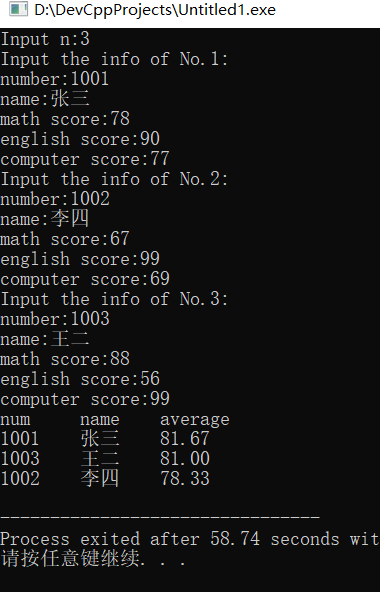修改学生成绩
输入n(n<=50)个学生的成绩信息，再输入一个学生的学号、课程以及成绩，在自定义函数中修改该学生指定课程的成绩
#include <stdio.h>
struct student
{
int num;
char name;
int computer,english,math;
double average;
};
int updata_score(struct student *p,int n,int num,int course,int score);
int main()
{
int course, i, n, num, pos, score;
struct student stu;
printf("Input n:");
scanf("%d",&n);
for(i=0;i<n;i++)
{
printf("Input the info of No.%d:\n",i+1);
printf("number:");
scanf("%d",&stu[i].num);
printf("name:");
scanf("%s",stu[i].name);
printf("math score:");
scanf("%d",&stu[i].math);
printf("english score:");
scanf("%d",&stu[i].english);
printf("computer score:");
scanf("%d",&stu[i].computer);
}
printf("Input the number of the students to be updata:");
scanf("%d",&num);
printf("Choise the course: 1.math  2.english  3.computer:");
scanf("%d",&course);
printf("Input the new score:");
scanf("%d",&score);
pos=updata_score(stu,n,num,course,score);
if(pos==-1)
else
{
printf("After updata:\n");
printf("num\tmath\tenglish\tcomputer\n");
printf("%d\t%d\t%d\t%d\n",stu[pos].num, stu[pos].math, stu[pos].english, stu[pos].computer);
}
return 0;
}
int updata_score(struct student *p,int n,int num,int course,int score)
{
int i,pos;
for(i=0;i<n;i++,p++)
if(p->num==num)
break;
if(i<n)
{
switch(course)
{
case 1:p->math=score;break;
case 2:p->english=score;break;
case 3:p->computer=score;break;
}
pos=i;
}
else
pos=-1;
return pos;
}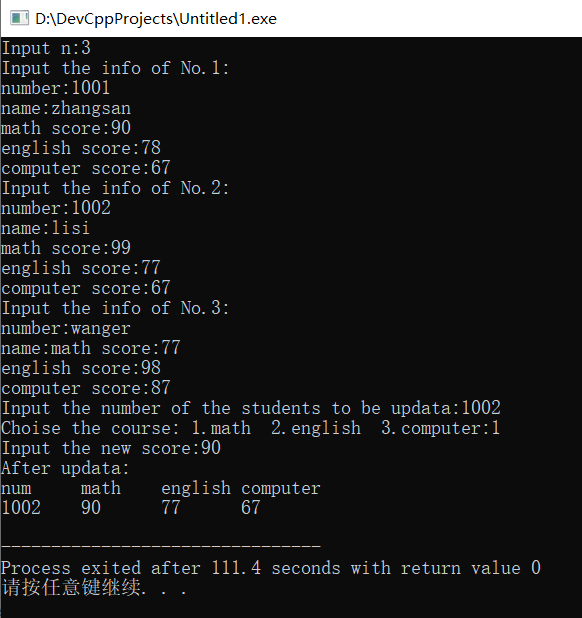下一篇文章
动态数组的实现，结合指针与结构体实现链表，以及链表相关操作
展开全文• 主要介绍了Go语言获取数组长度的方法,实例分析了len函数的使用技巧,具有一定参考借鉴价值,需要的朋友可以参考下
• ## C语言结构体数组

千次阅读 多人点赞 2018-10-18 19:50:54
C语言结构体数组 所谓结构体数组，是指数组中的每个元素都是一个结构体。在实际应用中，结构体数组常被用来表示一个拥有相同数据结构的群体，比如一个班的学生、一个车间的职工等。 定义结构体数组和定义结构体...
• C语言基础：求数组成员列表中的最大值并按序输出 例1： #include "stdafx.h" #include <stdio.h> #define member 5//成员数 #define NAME_LEN 20//姓名最大长度 struct st { int id; char name[NAME_LEN];...c语言 编程语言
• 结构体数组的初始化与前面讲的数值型数组的初始化是一模一样的，数值类型数组初始化的方法需要注意的是在结构体数组的初始化中同样适用，因为不管是不是数值型数组还是结构体数组都是数组。 #include<stdio.h>...
• 一、结构体指针 与一般指针类似结构体也可以使用结构体指针进行引用使用。结构体指针的定义方式如下： struct Student stu = { 1001,"Li Lei",'M',1.87 }; //定义一个Student 的结构体变量stu struct Student *p...
• C语言结构体数组概述 C语言定义结构体数组 C语言使用结构体数组注意事项 实例应用 C语言结构体数组概述 一个结构体变量中可以存放一组有关联的数据，如一个学生的学号、姓名、成绩等数据，如果有10个学生的...c语言 数据结构
• 一般情况下，对结构体类型的变量或者结构体类型的数组的赋值都是先定义一个结构体类型的变量，然后在对其属性进行赋值。例如： typedef struct Pi { int x; int y; } Pc; int main() { int i; Pc pc; pc[0...C语言
• #include #include typedef struct S_MenuFun { int id; void (*fun)(); }S_MenuFun; void fun1() { printf("fun1\n"); } void fun2() { printf("fun2\n"); } void fun3() {c语言
• ## C#结构体数组

千次阅读 2020-06-11 09:36:25
1．结构体类型的数组 ...结构体数组名=new 结构体类型名[数组长度]; 例如，定义包括100个点的结构体数组的代码如下： POINT[] p=new POINT; 或 POINT[] p=new POINT; POINT[] p; p=new
• 结构体是一种集合，它里面包含了多个变量或数组，它们的类型可以相同，也可以不同，每个这样的变量或数组都称为结构体的成员（Member）。请看下面的一个例子： struct stu{ char name[]; //姓...C语言
• ## C语言--结构体数组和结构体指针

万次阅读 多人点赞 2018-08-16 21:06:49
结构体是我们自己定义的一种新的数据类型, 同样可以有结构数组和结构指针。  1.结构体数组 具有相同结构体类型的变量构成的数组...}数组名[数组长度]; 或者： 先声明一个结构体类型，然后再定义结构体数组 结构...
• c语言 结构体数组的赋值 //**创建结构体** #include<stdio.h> struct Class{ ... //定义结构体数组长度所需变量 int i; printf("list size is : "); //定义结构体数组长度 scanf("%d",&m);c语言 数据结构
• C语言结构体数组初始化 一、使用场景 在C编码中，经常需要对结构体数组进行初始化。 使用这种方法，能够使得代码更加的清晰，易读。 例如：需要根据返回码，一个status code 做不同的操作。 int process_by_status...
• 定义一个结构体数组，为该数组开辟一块大的存储空间，然后进行初始化赋值。 #include<iostream> using namespace std; struct student { int ge; int gi; int b = { 0 }; }; int main...c++
• 结构体这块本来学着没有什么问题的，但是，有时候的学习不知道怎么的，可能是课程进度有点快...以至于在学到结构体数组时，比如：pArr为一个结构体数组，pArr.age等价于(*pArr).age，我一时脑回路不够用了，困惑...c语言自学 结构体指针变量
• 使用结构体数组 1. 定义结构体数组 栗子1： 有三个候选人，每个选民只能投票选一人，要求编一个统计选票的程序，先输入被选人的名字，最后输出各人得票结果 #include<stdio.h> #include<string.h>...C语言
• C语言结构体数组概述一个结构体变量中可以存放一组有关联的数据，如一个学生的学号、姓名、成绩等数据，如果有10个学生的数据需要参加运算，显然应该用数组，这就是结构体数组结构体数组与之前介绍...指针 golang 数据可视化 编程语言 hash
• 结构体数组 在C语言中我们可以通过struct关键字定义结构类型，结构中的字段占据连续的内存空间，每个结构体占用的内存大小都相同，因此可以很容易地定义结构数组。和C语言一样，在NumPy中也很容易对这种结构数组进行...python numpy
• ## C语言中的结构体数组

万次阅读 多人点赞 2017-01-15 00:45:10
C语言中的结构体数组1.结构体 数组概念 2. 结构体数组定义和初始化 3. 结构体数组的引用结构体数组的概念元素为结构体类型的数组称为结构体数组，在实际的应用过程中，经常使用结构体数组来表示具有相同数据结构...c语言 结构体 struct
• 目录 一、按照成员变量进行赋值（麻烦，好理解，字符串赋值需要strcpy） 二、对数组整体进行赋值。（一次性需要把所有的都添加进去，...（2）调用函数赋值，我们知道，结构体数组中，数组有多个元素，每个数组元素...c语言 开发语言 c++
• 文章目录结构体嵌套结构体数组初始化结构体数组结构体指针指针访问成员方法 结构体嵌套 比如上篇博客的代码 struct Book { char title; char author; float price; unsigned int date; char ...结构体指针
• 文章目录例题代码实现总结 例题 Input 第1排输入一个N，表示有N个学生的信息。(1&lt;=N&lt;=1000) 从第2排到第1+N排，每...每组测试数据中，所有学生的报名号的长度是一致的。初试成绩为2位的小数，范围在0...结构体 sort
• C#中结构体的定义时一些注意事项 本篇文章主要为记录使用结构体时遇到的一些问题，方便以后查找。 C#中定义结构体主要形式为 using System.Runtime.InteropServices; //结构体属性（一字节对齐），不写的话默认4...c#
• 结构体数组 在C语言中我们可以通过struct关键字定义结构类型，结构中的字段占据连续的内存空间，每个结构体占用的内存大小都相同，因此可以很容易地定义结构数组。和C语言一样，在NumPy中也很容易对这种结构数组进行...python
• C#:利用结构体数组完成简单信息输入、计算 using System; using System.Collections.Generic; using System.Linq; using System.Text; using System.Threading.Tasks; namespace norm_program { class Program { ...c#...